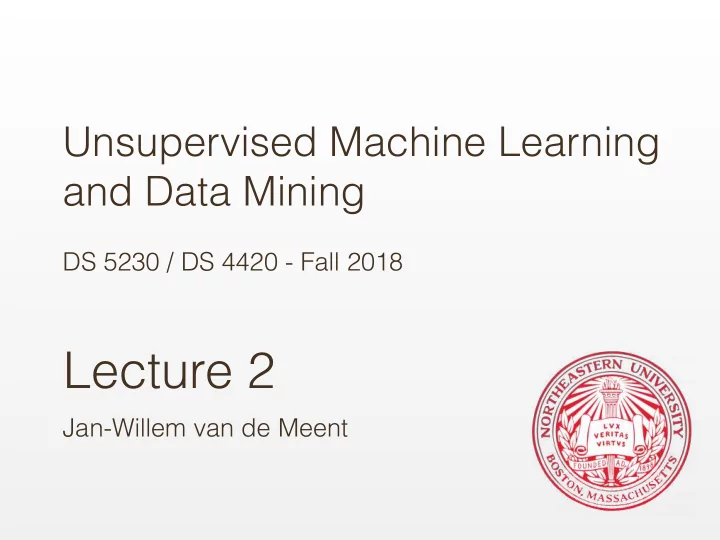# Lecture 2 Jan-Willem van de Meent Probability Examples: - PowerPoint PPT Presentation

## Unsupervised Machine Learning and Data Mining DS 5230 / DS 4420 - Fall 2018 Lecture 2 Jan-Willem van de Meent Probability Examples: Independent Events 1. Whats the probability of getting a sequence of 1,2,3,4,5,6 if we roll a dice six

1. Unsupervised Machine Learning   and Data Mining DS 5230 / DS 4420 - Fall 2018 Lecture 2 Jan-Willem van de Meent

2. Probability

3. Examples: Independent Events 1. What’s the probability of getting a sequence of 1,2,3,4,5,6 if we roll a dice six times? 2. A school survey found that 9 out of 10 students like pizza. If three students are chosen at random with replacement, what is the probability that all three students like pizza?

4. Dependent Events uit Apple or- intro- Orange Red bin Blue bin If I randomly pick a fruit from the red bin, what is the probability that I get an apple ?

5. Dependent Events uit Apple or- intro- Orange Red bin Blue bin Conditional Probability P(fruit = apple | bin = red ) = 2 / 8

6. Dependent Events uit Apple or- intro- Orange Red bin Blue bin Joint Probability P(fruit = apple , bin = red ) = 2 / 12

7. Dependent Events uit Apple or- intro- Orange Red bin Blue bin Joint Probability P(fruit = apple , bin = blue ) = ?

8. Dependent Events uit Apple or- intro- Orange Red bin Blue bin Joint Probability P(fruit = apple , bin = blue ) = 3 / 12

9. Dependent Events uit Apple or- intro- Orange Red bin Blue bin Joint Probability P(fruit = orange , bin = blue ) = ?

10. Dependent Events uit Apple or- intro- Orange Red bin Blue bin Joint Probability P(fruit = orange , bin = blue ) = 1 / 12

11. Two rules of Probability uit or- intro- 1. Sum Rule (Marginal Probabilities) P(fruit = apple ) = P(fruit = apple , bin = blue ) + P(fruit = apple , bin = red ) = ?

12. Two rules of Probability uit or- intro- 1. Sum Rule (Marginal Probabilities) P(fruit = apple ) = P(fruit = apple , bin = blue ) + P(fruit = apple , bin = red ) = 3 / 12 + 2 / 12 = 5 / 12

13. Two rules of Probability uit or- intro- 2. Product Rule P(fruit = apple , bin = red ) = P(fruit = apple | bin = red ) p(bin = red ) = ?

14. Two rules of Probability uit or- intro- 2. Product Rule P(fruit = apple , bin = red ) = P(fruit = apple | bin = red ) p(bin = red ) = 2 / 8 * 8 / 12 = 2 / 12

15. Two rules of Probability uit or- intro- 2. Product Rule (reversed) P(fruit = apple , bin = red ) = P(bin = red | fruit = apple ) p(fruit = apple ) = ?

16. Two rules of Probability uit or- intro- 2. Product Rule (reversed) P(fruit = apple , bin = red ) = P(bin = red | fruit = apple ) p(fruit = apple ) = 2 / 5 * 5 / 12 = 2 / 12

17. Bayes' Rule Posterior Likelihood Prior Sum Rule: Product Rule:

18. Bayes' Rule Posterior Likelihood Prior Probability of rare disease: 0.005 Probability of detection: 0.98 Probability of false positive: 0.05 Probability of disease when test positive?

19. Bayes' Rule Posterior Likelihood Prior 0.98 * 0.005 = 0.0049 0.98 * 0.005 + 0.05 * 0.995 = 0.0547 0.0049 / 0.0547 = 0.089

20.       Random Variables • Random Variable: A variable with a stochastic outcome   X = x x ∈ {1, 2, 3, 4, 5, 6} • Event: A set of outcomes   X >= 3 {3, 4, 5, 6} • Probability: The chance that a randomly selected   outcome is part of an event   P ( X >= 3 ) = 4 / 6

21.     Distribution • A distribution maps outcomes to probabilities   P ( X = x ) = 1 / 6 • Commonly used (or abused) shorthand:   P ( x ) is equivalent to P ( X = x )

More recommend